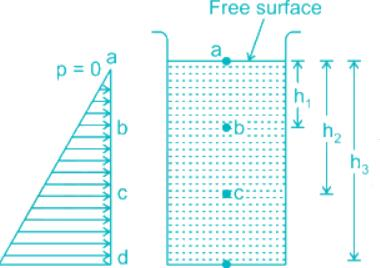Courses

# The depth of a fluid is measured in vertical Z – direction; X and Y are the other two directions and are mutually perpendicular. The static pressure variation in the fluid is given bya)dp/dz = gb)dp/dz = 0c)dp/dz = ρgd)dp/dz = −ρgCorrect answer is option 'D'. Can you explain this answer? Related Test: SSC JE (ME) Mock Test - 4

## SSC QuestionNaroj Boda Jul 25, 2021
The pressure at any point in a fluid at rest is obtained by the Hydrostatic Law, which states that the rate of increase of pressure in a vertically downward direction must be equal to the specific weight of the fluid at that point.p = ρgh
Pressure distribution profile
dp/dz = -ω = -pg
dp = -ω.dz
It indicates that a negative pressure gradient exists upward along any vertical.
Thus, the pressure decreases in the upward direction and increases in the downward direction with a magnitude equal to a specific weight.